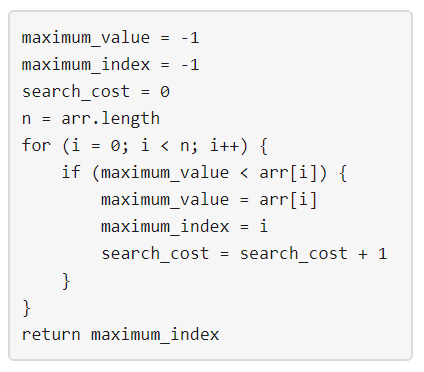# GeetCode Hub

Given three integers `n`, `m` and `k`. Consider the following algorithm to find the maximum element of an array of positive integers:You should build the array arr which has the following properties:

• `arr` has exactly `n` integers.
• `1 <= arr[i] <= m` where `(0 <= i < n)`.
• After applying the mentioned algorithm to `arr`, the value `search_cost` is equal to `k`.

Return the number of ways to build the array `arr` under the mentioned conditions. As the answer may grow large, the answer must be computed modulo `10^9 + 7`.

Example 1:

```Input: n = 2, m = 3, k = 1
Output: 6
Explanation: The possible arrays are [1, 1], [2, 1], [2, 2], [3, 1], [3, 2] [3, 3]
```

Example 2:

```Input: n = 5, m = 2, k = 3
Output: 0
Explanation: There are no possible arrays that satisify the mentioned conditions.
```

Example 3:

```Input: n = 9, m = 1, k = 1
Output: 1
Explanation: The only possible array is [1, 1, 1, 1, 1, 1, 1, 1, 1]
```

Example 4:

```Input: n = 50, m = 100, k = 25
Output: 34549172
Explanation: Don't forget to compute the answer modulo 1000000007
```

Example 5:

```Input: n = 37, m = 17, k = 7
Output: 418930126
```

Constraints:

• `1 <= n <= 50`
• `1 <= m <= 100`
• `0 <= k <= n`

class Solution { public int numOfArrays(int n, int m, int k) { } }